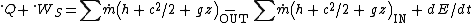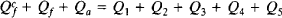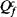Heat Balance

Heat balance

An application of the first law of thermodynamics to a process in which any work terms are negligible.

For a closed system, one that always consists of the same material, the first law is Q + W = ΔE, where Q is the heat supplied to the system, W is the work done on the system, and ΔE is the increase in energy of the material forming the system. It is convenient to treat ΔE as the sum of changes in mechanical energy, such as kinetic energy and potential energy in a gravitational field, and of internal energy ΔU that depends on changes in the thermodynamic state of the material. Because the rates at which any changes occur are usually of interest, heat balances are often written in terms of heat flow rates (heat per unit time), sometimes denoted by a dot over the symbol, , so that for a process with negligible work, kinetic energy and potential energy terms, , the rate of change of internal energy with time.

Often it is more convenient to apply the first law or a heat balance to an open system, a fixed region or control volume across the boundaries of which materials may travel and inside which they may accumulate, such as a building, an aircraft engine, or a section of a chemical process plant. Then the first law is expressed by the equation below, where is the rate of doing shaft workon the system; is the mass flow rate of any stream entering or leaving the control volume; h is the enthalpy per unit mass; c is the velocity; gz is the gravitational potential for each stream at the point of crossing the boundary of the control volume; and E is the energy of all material inside the control volume. When conditions inside the control volume do not change with time, although they need not be spatially uniform, dE/dt = 0, and the balance equation is known as the steady-flow energy equation.

Enthalpy is a thermodynamic property defined by h = u + pv, where u is the specific internal energy (enthalpy per unit mass), p the pressure, and v the specific volume. It is used, along with shaft work, because the derivation of the first-law equation for a control volume from the more fundamental equation for a closed system involves work terms pv that are not available for use outside the control volume. Changes in enthalpy occur because of changes in temperature, pressure, physical state (for example, from liquid to vapor), and changes in chemical state. See Enthalpy, Thermodynamic principles, Thermodynamic processes

McGraw-Hill Concise Encyclopedia of Physics. © 2002 by The McGraw-Hill Companies, Inc.
The following article is from The Great Soviet Encyclopedia (1979). It might be outdated or ideologically biased.

Heat Balance

a comparison of the heat received and expended (both usefully expended and lost) in various thermal processes. Heat balances are used in engineering for the analysis of thermal processes occurring in, for example, steam boilers, furnaces, and heat engines. A heat balance may be expressed in units of energy, such as joules or calories, or in percent of the total amount of heat per unit output, per hour of operation, per time period (cycle), or per kilogram of material used. In scientific research, heat balances are employed to solve many problems in, for example, astrophysics, geophysics, chemistry, and biology.

A heat balance is calculated on the basis of the enthalpies of the substances taking part in a process and the heats of the corresponding chemical reactions. For complex processes, especially in such areas as metallurgy and chemical engineering, the calculation of a heat balance precedes the calculation of a material balance, that is, a comparison of the masses of the input and output substances in the process. Here, the heat balance of a facility is often obtained as the sum of the heat balances of the pieces of equipment making up the facility. A distinction is made between rated heat balances and experimental heat balances, which are obtained from thermal testing data.Figure 1. Heat balance of a motor vehicle engine: (a) heat expended for useful purposes, (b) heat lost with exhaust gases, (c) heat lost to coolant, (d) other heat losses

A heat balance may be expressed in the form of an equation, with one side giving the sum of the amounts of input heat and the other side giving the amounts of heat used or lost. Heat balances may also be expressed in the form of a table or a diagram (Figure 1). The heat balance of a steam boiler, for example, can be expressed by the following equation:Here,is the heat of combustion of the fuel, Qf is the enthalpy of the fuel, Qa is the enthalpy of the air, Q1 is the neat transferred to the working medium, Q2 is the heat lost with the outgoing gases, Q3 and Q4 are the heat losses owing to chemical and mechanical underburning of the fuel, and Q5 is the heat lost owing to radiation.

A numerical value for the efficiency of a facility and of individual parts of a facility can be determined from heat balance data.

I. N. ROZENGAUZ

heat balance

[′hēt ‚bal·əns]
(geophysics)
The equilibrium which exists on the average between the radiation received by the earth and atmosphere from the sun and that emitted by the earth and atmosphere.
(metallurgy)
The calculation used in fluidization roasting so that the addition or removal of heat can be controlled to maintain the optimum temperature in the reacting vessel.
(thermodynamics)
The equilibrium which is known to exist when all sources of heat gain and loss for a given region or body are accounted for.
McGraw-Hill Dictionary of Scientific & Technical Terms, 6E, Copyright © 2003 by The McGraw-Hill Companies, Inc.

heat balance

1. A procedure for determining the efficiency of a combustion process: all heat losses (expressed as percentages) are added together; then their total is subtracted from 100%; the remaining figure represents the efficiency.
2. The establishment of a condition of thermal equilibrium in a space, wherein the heat gains just equal the heat losses.
McGraw-Hill Dictionary of Architecture and Construction. Copyright © 2003 by McGraw-Hill Companies, Inc.
References in periodicals archive ?
ANSYS thermal analysis module bases on heat balance equations derived by principle of conservation of energy, mainly involves steady-state analysis, transient analysis, thermal radiation, phase change, thermal stress and coupling field analysis related heat.
Heat balance analysis of the office space was carried out for summer and winter based on BEMS data.
This maximises productivity, whilst simultaneously ensuring the correct parameters were in place for heat balance and magnetohydrodynamic stability of the pots.
"If it is not thaw stable, then engineers either design the structure using specialized materials and construction techniques to keep the heat balance negative or build the structure in a way that it can be maintained as the permafrost thaws and settles."
Method for calculating the heat balance. The continuous operating temperature of the wire when the rated load current flows is determined on the basis of the heat balance equation between the extracted [P.sub.v] and the dissipated [P.sub.otd] power 
The practical application of the ASHRAE Heat Balance Method will often require computer software, especially considering the value of the time of the typical design engineer.
A heat gain model of occupants is deduced from the heat balance equation of the human body.
In the 1960s the late Ole Fanger, Ph.D., Fellow ASHRAE, proposed a heat balance model to predict the acceptability of a given thermal environment for a large group of people.
From outside the kiln, Exergen's sensor employs the heat balance technique to accurately detect the kiln's interior temperature, and both the Ovality and Exergen sensors send measurements to a personal computer using a Bluetooth connection.

Site: Follow: Share:
Open / Close